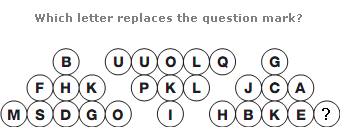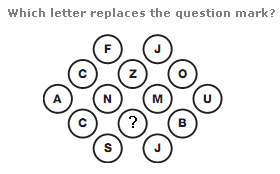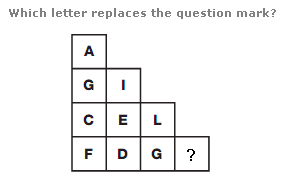# Puzzles - Missing letters puzzles

### Exercise :: Missing letters puzzlesAnswer : B Explanation : Add together the numerical values of the letters in corresponding positions on the left and right hand triangles, and put the letter with this value in corresponding segments of the centre triangle.Answer : A Explanation : Start on the centre left of the diagram, and move in a clockwise spiral towards the centre. Letters advance through the alphabet in steps of 2, 3, 4, etc.Answer : T Explanation : Working through the diagram in columns, left to right, The sum of the numerical values of the letters in each column follows the sequence 17, 18, 19 and 20.